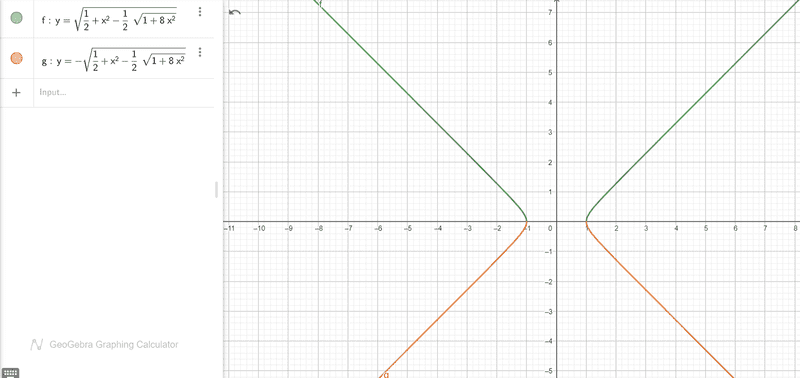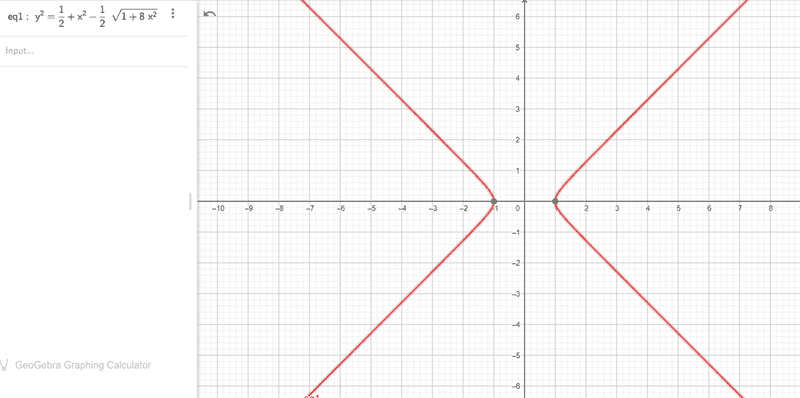# Find the range of ##k## for which ##\omega## is imaginary

• JD_PM
In summary, the given relation is a solution of the function ##\epsilon (k, \omega) = 1 - \frac{1}{2}(\frac{\omega_p^2}{(\omega-kv_0)^2} + \frac{\omega_p^2}{(\omega+kv_0)^2}) = 0##. To find the range of wave numbers ##k## for which the oscillation frequency ##\omega## is imaginary on the function, one can manipulate the equation to get ##\frac{(\omega^2+k^2v_0^2)}{(\omega^2-k^2v_0^2)^2} = \frac{1}{\omega_p^2

#### JD_PM

Homework Statement
Given the relation (treat ##k, \omega## as variables and ##v_0, \omega_p## as constants).

$$y^2=1+2\alpha^2 \pm(1+8\alpha^2)^{1/2}, \ \text{where} \ \alpha:= kv_0/\omega_p, \ y:= \sqrt{2}\omega/\omega_p$$

a) Find the range of wave numbers ##k## for which the oscillation frequency ##\omega## is imaginary

b) Find ##k_{max}## and ##\text{Im}(\omega_{max})##. Hint: sketch the solution for ##\text{Im}(\omega)##
Relevant Equations
N/A
Out of the given information I see no way of solving neither of the two sections.

So I did some reading and it turns out that the given relation is a solution of the following function (I won't prove it here)

$$\epsilon (k, \omega) = 1 - \frac 1 2 \left[ \frac{\omega_p^2}{(\omega-kv_0)^2} + \frac{\omega_p^2}{(\omega+kv_0)^2}\right] = 0$$

So I think that finding the range of wave numbers ##k## for which the oscillation frequency ##\omega## is imaginary on the ##\epsilon## function should be equivalent. I did some manipulations

$$\frac{\omega_p^2}{(\omega-kv_0)^2} + \frac{\omega_p^2}{(\omega+kv_0)^2} = 2 \Rightarrow \omega_p^2\left( \frac{\omega^2+2kv_0+k^2v_0^2+\omega^2-2kv_0+k^2v_0^2}{(\omega^2-k^2v_0^2)^2} \right) = 2$$

$$\frac{(\omega^2+k^2v_0^2)}{(\omega^2-k^2v_0^2)^2} = \frac{1}{\omega_p^2}$$

But got stuck in the above step...

I strongly think we better avoid using the function and focus on the given relation to answer the questions.

Might you please guide me through?

Thanks.

If ##y^2 < 0##, then y will be imaginary, and since ## y = \sqrt 2 \frac \omega {\omega_p}##, its seems likely that ##\omega## will also be imaginary, but without knowing whether ##\omega_p## is real, I can't say for sure.

Assuming for the moment that y being imaginary implies that ##\omega## will be imaginary, I'll continue on. Assuming that ##\alpha## is real, ##y^2 < 0## when ##1 + 2\alpha^2 - \sqrt{1 + 8\alpha^2} < 0##; i.e., when ##1 + 2\alpha^2 < \sqrt{1 + 8\alpha^2}## . Solving this inequality gives you a range of values for ##\alpha##, and presumably you can find a range of values for k.

•JD_PM
Let's assume ##\omega_p, k, v_0## to be real.

$$(1 + 2\alpha^2)^2 < 1 + 8\alpha^2 \Rightarrow \alpha^2 + \alpha^4 < 2\alpha^2 \Rightarrow \alpha^2(\alpha^2 - 1) < 0$$

Hence ##\alpha^2 < 0## or ##\alpha^2 < 1##. We assumed ##\omega_p, k, v_0## to be real so, by definition, ##\alpha## is real. Then, we stick to ##\alpha^2 < 0##.

Assuming ##v_0 \neq 0, \omega_p \neq 0##

$$k^4 < 0$$

Mmm but I expected to get a shorter range, I think I made a mistake somewhere.

JD_PM said:
Hence ##\alpha^2 < 0## or ##\alpha^2 < 1##. We assumed ##\omega_p, k, v_0## to be real so, by definition, ##\alpha## is real. Then, we stick to ##\alpha^2 < 0##.
If ##\alpha## is real, then its square can never be negative. On the other hand, ##\alpha^2 < 1 \Rightarrow -1 < \alpha < 1##.

•JD_PM
Mark44 said:
If ##\alpha## is real, then its square can never be negative.
That was my naïve mistake indeed! Thanks.

Hence the ##k## range is given by

$$-\left| \frac{\omega_p}{v_0}\right| < k < \left| \frac{\omega_p}{v_0}\right|$$

If you agree, let's tackle the next section

JD_PM said:
b) Find ##k_{max}## and ##\text{Im}(\omega_{max})##. Hint: sketch the solution for ##\text{Im}(\omega)##
About the hint: do they want us to sketch the imaginary solution for the relation

$$y^2=1+2\alpha^2 \pm(1+8\alpha^2)^{1/2}, \ \text{where} \ \alpha:= kv_0/\omega_p, \ y:= \sqrt{2}\omega/\omega_p$$

?

That is

$$0 > y^2=1+2\alpha^2 - (1+8\alpha^2)^{1/2}$$

With ##\omega## in the vertical axis and ##k## in the horizontal axis.

JD_PM said:
Hence the k range is given by $$-\left| \frac{\omega_p}{v_0}\right| < k < \left| \frac{\omega_p}{v_0}\right|$$

Given that ##y = \frac{\sqrt 2}{\omega_p}\omega##, then
##\left(\frac{\sqrt 2}{\omega_p}\omega \right)^2 = 1 + 2\alpha^2 - \sqrt{1 + 8\alpha^2}##
Solve for ##\omega## and then plug in some well-chosen values for ##\alpha##. That's what I would do. Note that due to the range of values for k, the graph should lie between the left- and right bounds for k shown above.

•JD_PM
Mark44 said:
Given that ##y = \frac{\sqrt 2}{\omega_p}\omega##, then
##\left(\frac{\sqrt 2}{\omega_p}\omega \right)^2 = 1 + 2\alpha^2 - \sqrt{1 + 8\alpha^2}##
Solve for ##\omega## and then plug in some well-chosen values for ##\alpha##.
We have

$$\omega = \pm \sqrt{\frac{\omega_p^2}{2} + \omega_p^2 \alpha^2 - \frac{\omega_p^2}{2} \sqrt{1 + 8\alpha^2} }$$

We know that ##-1 < \alpha < 1##, so I plotted the ##\omega## solution (y axis ##\omega##, x-axis ##\alpha##; I set ##\omega_p:= 1## for simplicity)Mark44 said:
Note that due to the range of values for k, the graph should lie between the left- and right bounds for k shown above.

Mmm I am doing something wrong, because within the left- and right bounds for ##k## I get nothing...

I thought of plugging special values for ##\alpha##, such as ##\alpha = 1##, but I get the trivial solution ##\omega = 0## ofc...

Mark44 said:
##\left(\frac{\sqrt 2}{\omega_p}\omega \right)^2 = 1 + 2\alpha^2 - \sqrt{1 + 8\alpha^2}##
It's probably better to solve for ##\omega^2##, and plot ##\omega^2## (vertical axis) vs. ##alpha## (horizontal axis). Remember my assumption that ##\omega## would be imaginary.

Here are some values I got in Excel, with ##\alpha## in the first column, and ##\omega^2## in the second column. I don't know the value for ##\omega_p##, so I just used 1, which seems to be what you did, as well.

 -0.9​ -0.05748​ -0.8​ -0.09693​ -0.7​ -0.11905​ -0.6​ -0.12489​ -0.5​ -0.11603​ -0.4​ -0.09498​ -0.3​ -0.06574​ -0.2​ -0.03446​ -0.1​ -0.00962​ -1.4E-16​ 0​ 0.1​ -0.00962​ 0.2​ -0.03446​ 0.3​ -0.06574​

•JD_PM
Mark44 said:
It's probably better to solve for ##\omega^2##, and plot ##\omega^2## (vertical axis) vs. ##alpha## (horizontal axis). Remember my assumption that ##\omega## would be imaginary.

.This is what I get for the ##\omega^2## (vertical axis) vs. ##alpha## (horizontal axis) plot (with ##\omega_p:= 1##)However, the hyperbolic behavior of the plot does not match your excel data...

Given that we are interested in plotting ##\text{Im}(\omega)## to eventually find ##k_{max}## and ##\text{Im}(\omega_{max})##, wouldn't be best to plot ##\omega## instead of ##\omega^2##? I would say that if we were to plot the former then ##\text{Im}(\omega)## would simply correspond to the ##-1 < \alpha < 1## range. Then, to find ##\text{Im}(\omega_{max})## we would just have to look at the maximum value of ##\omega## in that range and ##k_{max}## would be equivalent to find ##\alpha_{max}## (by definition of ##\alpha##).

JD_PM said:
Hence ##\alpha^2 < 0## or ##\alpha^2 < 1##.
Which I explained by saying that the first inequality couldn't occur for any real ##\alpha##, and the second one meant that ##-1 < \alpha < 1##.

JD_PM said:
However, the hyperbolic behavior of the plot does not match your excel data..
Sure it does. In the spreadsheet I was interested only in values of ##\alpha## in the interval (-1, 1). For these values, the corresponding values of ##\omega^2## are negative, hence the values of ##\omega## are imaginary. Think back to the title you chose for this thread.

Do you see the following plot OK? (with the axis as mentioned)
JD_PM said:
.This is what I get for the ##\omega^2## (vertical axis) vs. ##alpha## (horizontal axis) plot (with ##\omega_p:= 1##)

View attachment 295082

Mark44 said:
Sure it does. In the spreadsheet I was interested only in values of ##\alpha## in the interval (-1, 1). For these values, the corresponding values of ##\omega^2## are negative, hence the values of ##\omega## are imaginary. Think back to the title you chose for this thread.

JD_PM said:
Do you see the following plot OK? (with the axis as mentioned)
Yes, I saw the plot in that post. The part between the two parts of the hyperbola is precisely the part where ##\omega## is imaginary, which is what you asked about at the start of this thread.

JD_PM said:
Do you see the following plot OK? (with the axis as mentioned)

The spreadsheet values that @Mark44 posted are for ##\omega^2## versus ##\alpha##, whereas it appears that your graphing package takes the square root of the values it calculates for ##\omega^2## and plots that versus ##\alpha##.

•JD_PM
Hey guys, sorry for the late reply. After some thoughts and discussion with a smart colleague we got an answer!The plots are not required to solve the exercise, are just suggested. Let's try to avoid them for now.

JD_PM said:
b) Find ##k_{max}## and ##\text{Im}(\omega_{max})##. Hint: sketch the solution for ##\text{Im}(\omega)##

To find ##k_{max}## we can simply take ##\frac{d y}{d \alpha} = 0##. This leads to ##\alpha_{max} \pm \frac{2 \alpha_{max}}{\sqrt{1 + 8 \alpha_{max}^2}} = 0 \Rightarrow \alpha_{max} = \frac{\sqrt{6}}{4}##. We have defined ##\alpha:= kv_0/\omega_p##, so it follows that ##k_{max} = \frac{\sqrt{6}}{4} \frac{\omega_p}{v_0}##

Let's find ##\text{Im}(\omega_{max})##. We already know that ##\alpha_{max} = \frac{\sqrt{6}}{4}##. We have defined ##y:= \sqrt{2}\omega/\omega_p##. Hence plugging ##\alpha_{max}## into ##y##

\begin{align*} \text{Im}(\omega_{max}) &= \pm \frac{\omega_p}{\sqrt{2}}\sqrt{1 + 2\alpha_{max}^2 \pm \sqrt{1 + 8\alpha_{max}^2}} \\ &= \pm \frac{\omega_p}{\sqrt{2}} \sqrt{1 + \frac 3 4 \pm 2} \\ &= \pm \frac{\omega_p}{2} \sqrt{\frac{15}{2}} \end{align*}

Where I have used the fact that the imaginary part of a complex number is real.

Let me know your thoughts on the solution if you wishJD_PM said:
Hey guys, sorry for the late reply. After some thoughts and discussion with a smart colleague we got an answer!The plots are not required to solve the exercise, are just suggested. Let's try to avoid them for now.

To find ##k_{max}## we can simply take ##\frac{d y}{d \alpha} = 0##. This leads to ##\alpha_{max} \pm \frac{2 \alpha_{max}}{\sqrt{1 + 8 \alpha_{max}^2}} = 0 \Rightarrow \alpha_{max} = \frac{\sqrt{6}}{4}##. We have defined ##\alpha:= kv_0/\omega_p##, so it follows that ##k_{max} = \frac{\sqrt{6}}{4} \frac{\omega_p}{v_0}##

Let's find ##\text{Im}(\omega_{max})##. We already know that ##\alpha_{max} = \frac{\sqrt{6}}{4}##. We have defined ##y:= \sqrt{2}\omega/\omega_p##. Hence plugging ##\alpha_{max}## into ##y##

\begin{align*} \text{Im}(\omega_{max}) &= \pm \frac{\omega_p}{\sqrt{2}}\sqrt{1 + 2\alpha_{max}^2 \pm \sqrt{1 + 8\alpha_{max}^2}} \\ &= \pm \frac{\omega_p}{\sqrt{2}} \sqrt{1 + \frac 3 4 \pm 2} \\ &= \pm \frac{\omega_p}{2} \sqrt{\frac{15}{2}} \end{align*}

Where I have used the fact that the imaginary part of a complex number is real.

Let me know your thoughts on the solution if you wishNot quite right.

Remember, ##y##, and thus ##\omega##, is imaginary only when

##1 + 2\alpha_{max}^2 \pm \sqrt{1 + 8\alpha_{max}^2} < 0 ##

That happens only for the minus, not the plus, of the "##\pm##" and if ##0<\alpha^2<1##.

Added in Edit 40 minutes later:

I think you will also find that ##\dfrac{d y}{d \alpha} = 0## only for minus, not for the plus.
... except, of course, at ##\alpha =0##.

Last edited:
In particular, notice that ##\sqrt{1 + \dfrac 3 4 + 2} = \sqrt{\dfrac{15}{2}}## is a real number,

whereas ##\sqrt{1 + \dfrac 3 4 - 2} = \sqrt{\dfrac{-1}{4}} = \dfrac{1}{2} i \ ## is imaginary.

Last edited:
•JD_PM